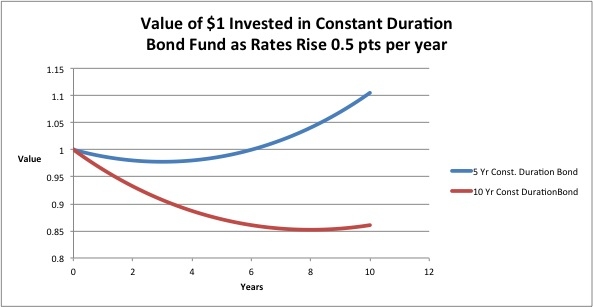Re Felix Salmons’s post on Whither Bond Returns, who asks: I would like to see a thought-through explanation of how and why I shouldn’t be too worried about entering what is sure to be a long-term period of rising interest rates.

Here is a simulation of what will happen to an investment in a bond fund when rates rise at a steady rate. It shows that you should be worried.

I assume an initial investment of \$1 in a par bond with a constant duration. The bond is issued at par, starting with a yield of 1% (for example).

Then I assume rates rise steadily at 0.5 percentage points per year.

Each month, the bond spins off a cash coupon, positive, but declines in value due to the rise in yields. At the end of each month, the bond is sold and together with the coupon, the money received is reinvested in a new bond with the same duration as the original one.Here is the formula: Value(t) = exp((y_0-Nk)*t+0.5kt^2). It assumes everything is done continuously.

Key: t = time

y_0 = initial yield (where 1% is entered as 0.01

N = duration in years

k = increase per year in yields (so an increase of 1/2 a point per years means k = 0.005).

As you can see, a 5-year duration bond declines in value but eventually starts to recover and produce positive returns.

It takes much longer for a 10-year duration bond.

The time to get back to par is 2N – 2y/k, so the faster rates rise (k increases) the longer it takes to get back to par and then above it.

_______

Here is the WolframAlpha code for plotting the function easily (though the graphs above weren’t produced that way):

plot exp((y – N k)*t+0.5k t^2) for k = 0.01, N=5, y=0.03, for t = 0 to 1

Now can modify the parameters interactively

___________

Another graph:Published in other